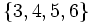# Automorph-conjugacy is not finite-conjugate-intersection-closed

This article gives the statement, and possibly proof, of a subgroup property (i.e., automorph-conjugate subgroup) not satisfying a subgroup metaproperty (i.e., finite-conjugate-intersection-closed subgroup property).
View all subgroup metaproperty dissatisfactions | View all subgroup metaproperty satisfactions|Get help on looking up metaproperty (dis)satisfactions for subgroup properties
Get more facts about automorph-conjugate subgroup|Get more facts about finite-conjugate-intersection-closed subgroup property|

## Statement

We can have a group$G$ with two automorph-conjugate subgroups$H, K \le G$, such that$H$ and$K$ are conjugate subgroups within$G$, and$H \cap K$ is not automorph-conjugate in$G$.

## Proof

### Example in the symmetric group

Further information: symmetric group:S6

Let$G$ be the symmetric group on six letters:$\{ 1,2,3,4,5,6 \}$. Let$H, K$ be the following 2-Sylow subgroups of$G$:$H = \langle (1,3,2,4) , (1,2), (5,6) \rangle ;\qquad K = \langle (1,2), (3,5,4,6), (5,6) \rangle$

In other words,$H$ is the internal direct product of a 2-Sylow subgroup on$\{ 1,2,3,4\}$ with the 2-Sylow subgroup on$\{ 5,6\}$, while$K$ is the internal direct product of the 2-Sylow subgroup on$\{ 1,2 \}$ with a 2-Sylow subgroup on$\{ 3,4,5,6 \}$.

The intersection is given by:$H \cap K = \langle (1,2), (3,4), (5,6) \rangle$.

Now, note that:

• Both$H$ and$K$ are automorph-conjugate, because they are both Sylow subgroups, and Sylow implies automorph-conjugate. Moreover, they are conjugate to each other, since Sylow implies order-conjugate.
•$H \cap K$ is not automorph-conjugate. To see this, note that$G$ has an outer automorphism that sends transpositions to triple transpositions. Under this automorphism,$H \cap K$ goes to a subgroup of$G$ that contains three commuting triple transpositions. If this is conjugate to$H \cap K$, then$H \cap K$ should also contain three commuting triple transpositions. But it doesn't.